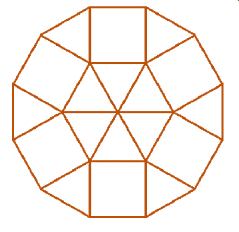# C++ program to count number of dodecagons we can make of size d

Suppose we have a number d. Consider there is an infinite number of square tiles and regular triangular tiles with sides length 1. We have to find in how many ways we can form regular dodecagon (12-sided polygon) with sides d using these tiles. If the answer is too large, return result mod 998244353.## Steps

To solve this, we will follow these steps−

b := floor of d/2 - 1
c := 1
for initialize i := 2, when i < d, update (increase i by 1), do:
b := b * (floor of d/2)
c := c * i
return (b / c)

## Example

Let us see the following implementation to get better understanding −

#include <bits/stdc++.h>
using namespace std;

int solve(int d){
int b = ((d << 1) - 1);
int c = 1;
for (int i = 2; i < d; i++){
b *= (d << 1) - i;
c *= i;
}
return (b / c);
}
int main(){
int d = 1;
cout << solve(d) << endl;
}

## Input

1

## Output

1